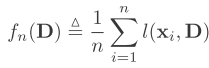## 2012年3月21日 星期三

### Online Dictionary Learning for Sparse Coding

We know that sparse coding is one of the most used methods to quantize the features.
And the performance of sparse coding is highly related to the codebook (centroids) it uses.
Therefore, this paper want to provide a efficient method to learn the codebook well.

They said there are three main contributions in this paper
●Cast the dictionary learning problem into optimization problem, which minimizes the expected cost
●Propose a online learning algorithm
●The algorithm is significantly faster than previous algorithms

Before introducing their algorithm, let us first introduce the problem definition of sparse coding.
Given a set of signals X= [X1, ..., Xn], it want to minimizewhere D is the learned dictionary (codebook). l is a function to measure the distance between original signal and quantized signal. That is, the quantization error. We not only want to minimize this function, but also want quantized signals to be sparse.Thus, there is a L1 term in the l functionHere we want to minimize the value of alpha. However, we can arbitrarily increase the elements in D to decrease the value of alpha. Thus, we have to constrain the value of elements in D.
The L2-norm of each column in D should be less than 1.
Finally, the problem becomes that
We need to solve two items in this equation. One is D, and the other is alpha.
It is quite hard to solve these two variables at the same. Therefore, we often solve one variable first by fixing another variable, and then solve another iteratively.

The following is the main algorithm of this paper
As we can see, it first solve alpha by using the D in previous iteration, and then solve D by fixing the alpha.

But learning a new dictionary for every input signal Xi is not efficient.
Instead, they train a new dictionary after collecting Eta signals, and using different updating methods as follow.
Experiments:
They compared the results of this online method with Batch method and the Stochastic Gradient Descent.
Objective function is fn(D),which is also known as quantization error. Thus, the smaller is the better.
We can found that the performance of online-learning is better than batch methods, and is almost the same as Stochastic Gradient Descent.
Although the performance is almost the same, Stochastic Gradient Descent needs to tune the learning rate Rho.

========================================================
Comment:
This paper describes a useful online codebook learning method. Although it has the term "Sparse Coding" in the title, it doesn't focus on this. It main focus on "Online learning".
They provide a very detail theoretical prove to convince the readers it is not just an ad-hoc method. That is quite important in this paper, but hard to read.
Furthermore, they said they have three contributions, but I think the first one is not the contribution from them.